Courses

# RD Sharma Solutions Ex-2.1, Exponents Of Real Numbers, Class 9, Maths Class 9 Notes | EduRev

## RD Sharma Solutions for Class 9 Mathematics

Created by: Abhishek Kapoor

## Class 9 : RD Sharma Solutions Ex-2.1, Exponents Of Real Numbers, Class 9, Maths Class 9 Notes | EduRev

The document RD Sharma Solutions Ex-2.1, Exponents Of Real Numbers, Class 9, Maths Class 9 Notes | EduRev is a part of the Class 9 Course RD Sharma Solutions for Class 9 Mathematics.
All you need of Class 9 at this link: Class 9

Q.1. Simplify the following:

(i) 3(a4 b3)10 x 5 (a2 b2)3

Solution: = 3(a40 b30) x 5 (a6 b6) = 15 (a46 b36

(ii) (2x -2 y3)3

Solution:  = (23 x -2x3 y3x3) = 8x -6 y9

(iii)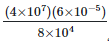Solution: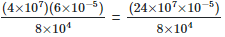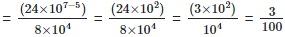(iv)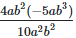Solution: =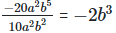(v)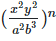Solution: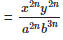(vi)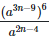Solution: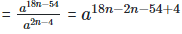= a16n−50

2. If a = 3 and b = -2, find the values of:

(i) aa+bb
(ii) ab+ba
(iii) ab+ba

Solution: (i) We have,aa+bb = 33+(−2)−2 = 33+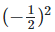= 27+1/4 = 109/4

(ii) ab+ba = 3−2+(−2)3 = (1/3)2+(−2)3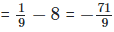(iii) We have, ab+ba = (3+(−2))3(−2) = (3−2))−6 = 1−6 = 1

3.Prove that: (i)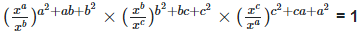(ii)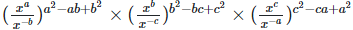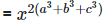(iii)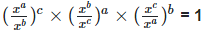Solution: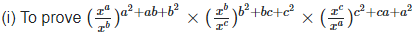= 1

Left hand side (LHS) = Right hand side (RHS) Considering LHS,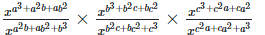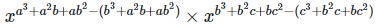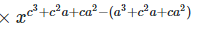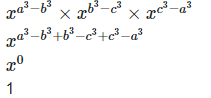Or, Therefore, LHS = RHS Hence proved

(ii) To prove,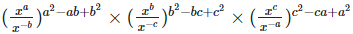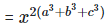Left hand side (LHS) = Right hand side (RHS) Considering LHS,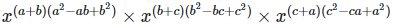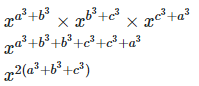Therefore, LHS = RHS Hence proved (iii) To prove,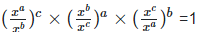Left hand side (LHS) = Right hand side (RHS) Considering LHS,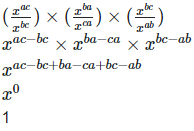Therefore, LHS = RHS Hence proved

4. Prove that: (i)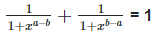(ii)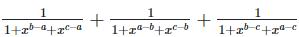Solution: (i)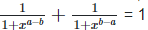Left hand side (LHS) = Right hand side (RHS) Considering LHS,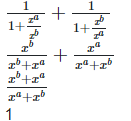Therefore, LHS = RHS Hence proved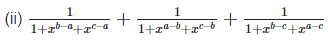Left hand side  (LHS) = Right hand side (RHS) Considering LHS,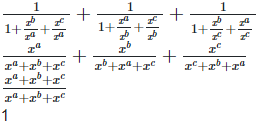Therefore, LHS = RHS Hence proved

5.Prove that: (i)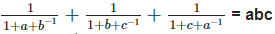(ii)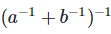Solution: (i) To prove,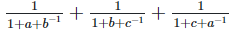= abc

Left hand side (LHS) = Right hand side (RHS) Considering LHS,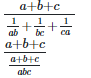abc

Therefore, LHS = RHS Hence proved (ii) To prove,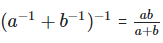Left hand side (LHS) = Right hand side (RHS) Considering LHS,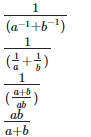Therefore, LHS = RHS Hence proved

6. If abc = 1, show that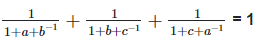Solution: To prove,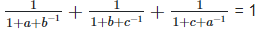Left hand side (LHS) = Right hand side (RHS) Considering LHS,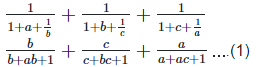We know abc = 1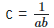By substituting the value c in equation (1), we get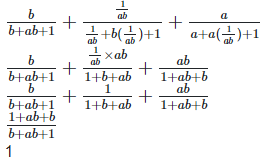Therefore, LHS = RHS Hence proved

7. Simplify: (i)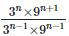Solution: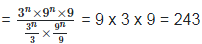(ii)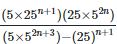Solution: =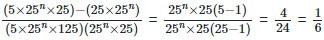(iii)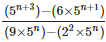Solution: =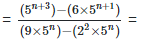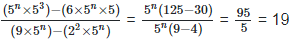(iv)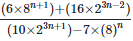Solution: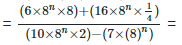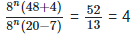8. Solve the following equations for x:

(i) 72x+3 = 1
(ii) 2x+1=4x−3
(iii) 25x+3 = 8x+3
(iv) 42x = 13/2
(v) 4x−1×(0.5)3−2x=(1/8)x
(vi) 23x−7 = 256

Solution: (i) We have,

⇒ 72x+3 = 1 ⇒ 72x+3 = 70 ⇒ 2x + 3 = 0 ⇒ 2x = -3 ⇒ x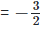(ii) We have,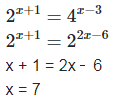(iii) We have,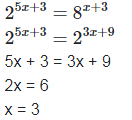(iv) We have,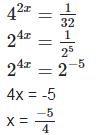(v) We have,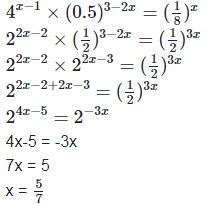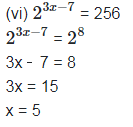9. Solve the following equations for x:

(i) 22x−2x+3+24 = 0

(ii) 32x+4+1=2×3x+2

Solution:

(i) We have, ⇒ 22x−2x+3+24 = 0

⇒  22x+24=2x.23
⇒ Let 2x=y
⇒ y2+24=y×23
⇒  y2−8y+16=0
⇒ y2−4y−4y+16=0
⇒ y(y-4) -4(y-4) = 0
⇒ y = 4
⇒ x2=22
⇒ x = 2

(ii) We have,

32x+4+1=2×3x+2

(3x+2)2+1=2×3x+2

Let 3x+2 = y

y2+1=2y

y2−2y+1=0

y2−y−y+1=0

y(y−1)−1(y−1)=0

(y−1)(y−1)=0

y = 1

10. If 49392 = a4b2c3, find the values of a, b and c, where a, b and c, where a, b, and c are different positive primes.

Solution: Taking out the LCM , the factors are 24,3and 73 a4b2c3 = 24,3and 73

a = 2, b = 3 and c = 7 [Since, a, b and c are primes]

11. If 1176 = 2a×3b×7c, Find a, b, and c.

Solution: Given that 2, 3 and 7 are factors of 1176. Taking out the LCM of 1176, we get 23×31×72 = 2a×3b×7c By comparing, we get

a = 3, b = 1 and c = 2.

12. Given 4725 = 3a×5b×7c, find (i) The integral values of a, b and c

Solution: Taking out the LCM of 4725, we get

33×52×71 = 3a×5b×7c

By comparing, we get

a = 3, b = 2 and c = 1.

(ii) The value of 2−a×3b×7c

Solution:

= 2−3×32×71

2−3×32×71 = 1/8×9×7

63/8

13. If a = xyp−1, b = xyq−1 and c = xyr−1, prove that aq−rbr−pcp−q = 1

Solution: Given, a = xyp−1, b = xyq−1 and c = xyr−1

To prove, aq−rbr−pcp−q = 1

Left hand side (LHS) = Right hand side (RHS) Considering LHS, = aq−rbr−pcp−q        ……(i)

By substituting the value of a, b and c in equation

(i), we get

= (xyp−1)q−r(xyq−1)r−p(xyr−1)p−q

= xypq−pr−q+rxyqr−pq−r+pxyrp−rq−p+q

= xypq−pr−q+r+qr−pq−r+p+rp−rq−p+q

= xy0 = 1

Offer running on EduRev: Apply code STAYHOME200 to get INR 200 off on our premium plan EduRev Infinity!

91 docs

,

,

,

,

,

,

,

,

,

,

,

,

,

,

,

,

,

,

,

,

,

,

,

,

,

,

,

,

,

,

;